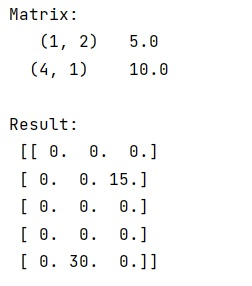# Elementwise multiplication of a scipy.sparse matrix by a broadcasted dense 1d array

Learn, how to elementwise-multiply a scipy.sparse matrix by a broadcasted dense 1d array in Python?
Submitted by Pranit Sharma, on February 26, 2023

NumPy is an abbreviated form of Numerical Python. It is used for different types of scientific operations in python. Numpy is a vast library in python which is used for almost every kind of scientific or mathematical operation. It is itself an array which is a collection of various methods and functions for processing the arrays.

## Elementwise-multiplying a scipy.sparse matrix by a broadcasted dense 1d array

Scipy is an abbreviated form of Scientific Python. It is a scientific computation library that provides utility functions and offers more optimization and signal processing. Scipy is an open-source library that can be used freely. Scipy uses Numpy to run its added optimized functions in the field of Data Science.

The term broadcasting describes how NumPy treats arrays with different shapes during arithmetic operations. Broadcasting provides a means of vectorizing array operations so that looping occurs in C instead of Python.

Suppose that we are given a 2D sparse array and a dense 1D array with all the non-zero elements. We need to compute the elementwise multiplication of arr1 and dense array using the usual broadcasting semantics of numpy.

For this purpose, we can turn the vector into a sparse diagonal matrix and then use matrix multiplication (with *) to do the same thing as broadcasting.

Let us understand with the help of an example,

## Python code for elementwise multiplication of a scipy.sparse matrix by a broadcasted dense 1d array

```# Importing scipy sparse
import scipy.sparse as ssp

# Import numpy
import numpy as np

# Creating a scipy sparse matrix
mat = ssp.lil_matrix((5,3))

# Giving elements
mat[1,2] = 5
mat[4,1] = 10

# Display matrix
print("Matrix:\n",mat,"\n")

# Creating a dense array
den = ssp.lil_matrix((3,3))
den.setdiag(np.ones(3)*3)

# Multiplying array with dense array
# and converting it into dense matrix
res = (mat*den).todense()

# Display result
print("Result:\n",res)
```

Output:Languages: » C » C++ » C++ STL » Java » Data Structure » C#.Net » Android » Kotlin » SQL
Web Technologies: » PHP » Python » JavaScript » CSS » Ajax » Node.js » Web programming/HTML
Solved programs: » C » C++ » DS » Java » C#
Aptitude que. & ans.: » C » C++ » Java » DBMS
Interview que. & ans.: » C » Embedded C » Java » SEO » HR
CS Subjects: » CS Basics » O.S. » Networks » DBMS » Embedded Systems » Cloud Computing
» Machine learning » CS Organizations » Linux » DOS
More: » Articles » Puzzles » News/Updates